# Using Data Cubes## Overview

Last Update: 5 Feb 2010 - reviewed for CIAO 4.2: no changes

Synopsis:

The CIAO Data Model allows you to filter and manipulate 3-dimensional images, known as "data cubes." You can select 3-dimensional subsets, or slice out 2-dimensional pieces. If two dimensions of the cube represent a pair of position axes, you can apply a region filter to those axes.

Purpose:

To create and filter data cubes.

## Get Started

Sample ObsID used: 1463 (ACIS-S, Jupiter)

The thread also uses a VLA radio image from the NRAO Archive (project AZ0128).

## Creating a Position-time Data Cube from an Event File

While we choose to create an (x,y,time) data cube, users may bin on any three columns that make sense in the analysis. For instance, you may want to create a PHA or energy axis to see how the spectral characteristics of a source change over time.

A common analysis involving data cubes is to create a file with two position axes and one time axis. This example shows how one might choose the binning parameters to create such a cube by inspecting various possibilities via dmlist before writing out the file.

Here we are using an observation of jupiter - acisf01463N002_evt2.fits. We need to determine a suitable time range and step size, and select a spatial range for the filtering.

What if we were to simply bin all three axes by a factor of one? dmcopy can be used to examine the effects by creating a virtual output file:

```unix% dmlist "acisf01463N002_evt2.fits[bin x,y,time]" cols
# DMLIST (CIAO 4.2): WARNING: Creating large image: 1745 MB. Current max set at 50 MB.
Increase maximum using [opt mem=n] or increase blocking to reduce size.

--------------------------------------------------------------------------
Columns for Image Block EVENTS_IMAGE
--------------------------------------------------------------------------

ColNo  Name                 Unit        Type             Range
1   EVENTS_IMAGE[8192,8192,22381]              Int2(8192x8192x22381) -

--------------------------------------------------------------------------
Physical Axis Transforms for Image Block EVENTS_IMAGE
--------------------------------------------------------------------------

Group# Axis#
1   1,2    sky(x) = (#1) [pixel]
(y)   (#2)
2   3      time                 = +59969680.685274 [s] +1.0 * (#3  -0.50)

--------------------------------------------------------------------------------
World Coordinate Axis Transforms for Image Block EVENTS_IMAGE
--------------------------------------------------------------------------------

Group# Axis#
1   1,2    EQPOS(RA ) = (+24.6832) +TAN[(-0.000136667)* (sky(x)-(+4096.50))]
(DEC)   (+8.6784 )      (+0.000136667)  (   (y) (+4096.50))
```

The result is an 8192 x 8192 x 22381 cube, which would about 3 terabytes in size.

The time axes is 22381 pixels because the default pixel size is one second and this is a 22 ks observation. We can shorten this axis to only 22 steps in time by binning in units of 1000 seconds. Additionally, the spatial size can be reduced by binning x and y by a factor of 8:

```unix% dmlist "acisf01463N002_evt2.fits[bin x=::8,y=::8,time=::1000]" cols

---------------------------------------------------------------------
Columns for Image Block EVENTS_IMAGE
---------------------------------------------------------------------

ColNo  Name                 Unit        Type             Range
1   EVENTS_IMAGE[1024,1024,23]              Int2(1024x1024x23) -

---------------------------------------------------------------------
Physical Axis Transforms for Image Block EVENTS_IMAGE
---------------------------------------------------------------------

Group# Axis#
1   1,2    sky(x) = (+0.50)[pixel] +(+8.0)* ((#1)-(+0.50))
(y)   (+0.50)         (+8.0)  ((#2) (+0.50))
2   3      time                 = +59969680.685274 [s] +1000.0 * (#3  -0.50)

---------------------------------------------------------------------
World Coordinate Axis Transforms for Image Block EVENTS_IMAGE
---------------------------------------------------------------------

Group# Axis#
1   1,2    EQPOS(RA ) = (+24.6832) +TAN[(-0.000136667)* (sky(x)-(+4096.50))]
(DEC)   (+8.6784 )      (+0.000136667)  (   (y) (+4096.50))
```

The output is now a 1024 x 1024 x 23 cube, which is more reasonable.

An alternate approach is to keep the full spatial resolution, but use a small region of the file:

```unix% dmlist "acisf01463N002_evt2.fits[bin x=3900:4400,y=4100:4600,time=::1000]" cols

--------------------------------------------------------------------------------
Columns for Image Block EVENTS_IMAGE
--------------------------------------------------------------------------------

ColNo  Name                 Unit        Type             Range
1   EVENTS_IMAGE[500,500,23]              Int2(500x500x23) -

--------------------------------------------------------------------------------
Physical Axis Transforms for Image Block EVENTS_IMAGE
--------------------------------------------------------------------------------

Group# Axis#
1   1,2    sky(x) = (+3900.0)[pixel] +(+1.0)* ((#1)-(+0.50))
(y)   (+4100.0)         (+1.0)  ((#2) (+0.50))
2   3      time                 = +59969680.685274 [s] +1000.0 * (#3  -0.50)

--------------------------------------------------------------------------------
World Coordinate Axis Transforms for Image Block EVENTS_IMAGE
--------------------------------------------------------------------------------

Group# Axis#
1   1,2    EQPOS(RA ) = (+24.6832) +TAN[(-0.000136667)* (sky(x)-(+4096.50))]
(DEC)   (+8.6784 )      (+0.000136667)  (   (y) (+4096.50))
```

which shows that we will get a 500 x 500 x 23 image.

Finally, we decide to use this binning with dmcopy to create the file:

```unix% dmcopy "acisf01463N002_evt2.fits[bin x=3900:4400,y=4100:4600,time=::1000]" jupiter_cube.fits

unix% dmlist jupiter_cube.fits blocks

--------------------------------------------------------------------------------
Dataset: jupiter_cube.fits
--------------------------------------------------------------------------------

Block Name                          Type         Dimensions
--------------------------------------------------------------------------------
Block    1: EVENTS_IMAGE                   Image      Int2(500x500x23)
Block    2: GTI7                           Table         2 cols x 5        rows
Block    3: GTI0                           Table         2 cols x 3        rows
Block    4: GTI1                           Table         2 cols x 2        rows
Block    5: GTI2                           Table         2 cols x 3        rows
Block    6: GTI3                           Table         2 cols x 5        rows
```

As expected, the output is a 500 x 500 x 23 image.

### How to display the file

SAOImage ds9, the default imager distributed with CIAO, has the capability to display data cubes.

```unix% ds9 jupiter_cube.fits &
```

When the file is opened, ds9 automatically detects that it is a cube and launches the data cube dialog box, as shown in Figure 1. (If the data cube dialog box doesn't launch, open it from the "Frame" menu.) The spatial axes are displayed, while the third axis - time, in this case - is accessible via the dialog box. When "Play" is chosen, ds9 cycles through the bins of the time axis, essentially creating a movie of the object. The speed of the frame changes is controlled from the "Interval" menu of the dialog box.

### Figure 1: Viewing a data cube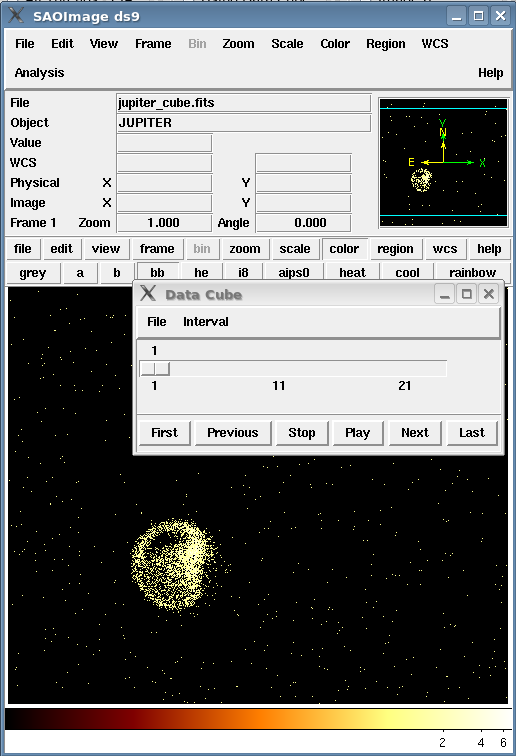### Figure 1: Viewing a data cube

This data cube has 23 intervals. Choosing "Play" from the data cube window steps through the intervals.

The other buttons in the dialog box (e.g. "Prev" and "Next") allow the user to move back and forth manually as well.

## Manipulating the Data Cube

The data cube can be filtered using DM syntax in the same way as 2D files. All of these examples use the data cube created in the previous section.

### Range filtering

A filter is applied to the time column of the cube to select a range of 3500 s:

```unix% dmcopy "jupiter_cube.fits[time=59969680:59973180]" range_cube.fits

unix% dmlist range_cube.fits cols

--------------------------------------------------------------------------------
Columns for Image Block EVENTS_IMAGE
--------------------------------------------------------------------------------

ColNo  Name                 Unit        Type             Range
1   EVENTS_IMAGE[500,500,4]              Int2(500x500x4) -

--------------------------------------------------------------------------------
Physical Axis Transforms for Image Block EVENTS_IMAGE
--------------------------------------------------------------------------------

Group# Axis#
1   1,2    sky(x) = (+3900.0) +(+1.0)* ((#1)-(+0.50))
(y)   (+4100.0)  (+1.0)  ((#2) (+0.50))
2   3      time                 = +59969680.685274 [s] +1000.0 * (#3  -0.50)

--------------------------------------------------------------------------------
World Coordinate Axis Transforms for Image Block EVENTS_IMAGE
--------------------------------------------------------------------------------

Group# Axis#
1   1,2    EQPOS(RA ) = (+24.6832) +TAN[(-0.000136667)* (sky(x)-(+4096.50))]
(DEC)   (+8.6784 )      (+0.000136667)  (   (y) (+4096.50))
```

The output file is 500 x 500 x 4, since the filter spanned four of the 1000 s time bins. Displaying the file in ds9 (Figure 2) looks similar to the unfiltered file; there are just fewer steps available in the data cube dialog box.

### Figure 2: Time-filtered data cube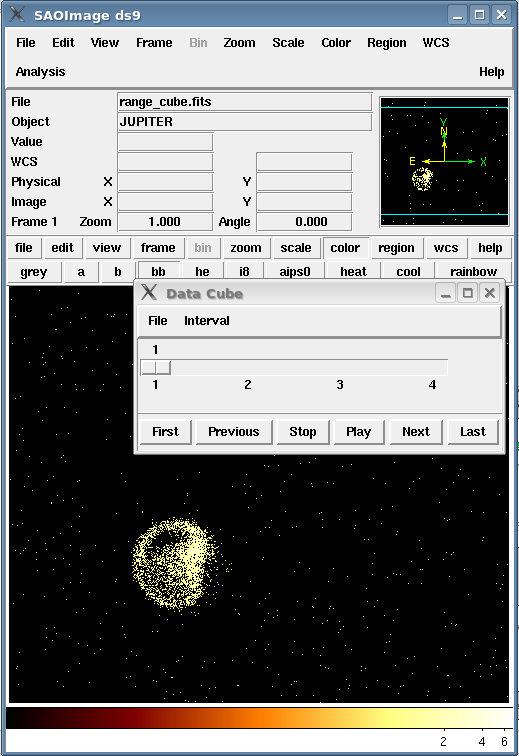### Figure 2: Time-filtered data cube

This data cube has 4 intervals.

### Region filtering

One may decide to apply a region filter to restrict the spatial axes of the file further. The region file used here, shown on the data in Figure 3 is:

```unix% cat circle.reg
# Region file format: CIAO version 1.0
circle(4143.5,4266.5,137.16489)
```

Note that if you are working with an object that moves in time, such as the solar system object we're using, make sure the region is large enough that the object won't drift out of the field of view. Define the region, then use the data cube dialog box to step forward and confirm that the object will still lie within the region.

### Figure 3: Region filter overlaid on the data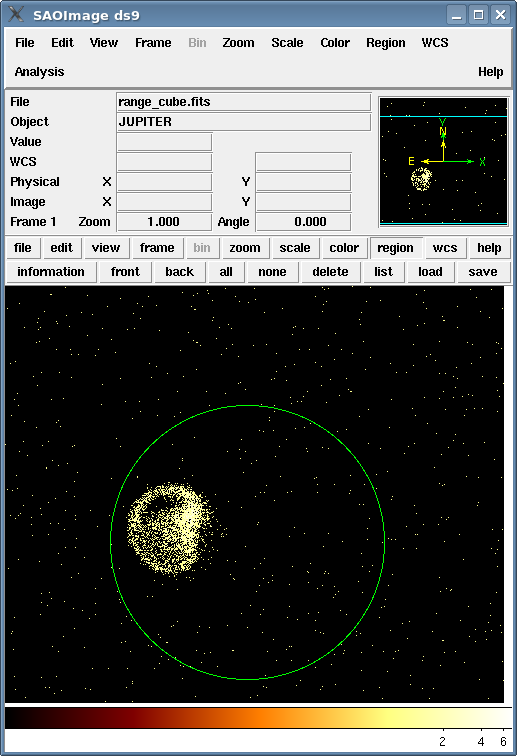### Figure 3: Region filter overlaid on the data

The region is large enough that Jupiter won't drift out of it over the time interval.

dmcopy is used to apply the region filter to the unfiltered data cube:

```unix% dmcopy "jupiter_cube.fits[sky=region(circle.reg)][opt null=-99]" region_cube.fits
```

Note that if you don't want to use a region file, the equivalent syntax for defining the region on the command line is:

```unix% dmcopy "jupiter_cube.fits[sky=circle(4143.5,4266.5,137.16489)][opt null=-99]" region_cube_2.fits
```

The resulting file has the same time axis as the unfiltered cube, but smaller spatial axes:

```unix% dmlist region_cube.fits cols

--------------------------------------------------------------------------------
Columns for Image Block EVENTS_IMAGE
--------------------------------------------------------------------------------

ColNo  Name                 Unit        Type             Range            Null
1   EVENTS_IMAGE[275,275,23]              Int2(275x275x23) -                    -99

--------------------------------------------------------------------------------
Physical Axis Transforms for Image Block EVENTS_IMAGE
--------------------------------------------------------------------------------

Group# Axis#
1   1,2    sky(x) = (+4006.0) +(+1.0)* ((#1)-(+0.50))
(y)   (+4129.0)  (+1.0)  ((#2) (+0.50))
2   3      time                 = +59969680.685274 [s] +1000.0 * (#3  -0.50)

--------------------------------------------------------------------------------
World Coordinate Axis Transforms for Image Block EVENTS_IMAGE
--------------------------------------------------------------------------------

Group# Axis#
1   1,2    EQPOS(RA ) = (+24.6832) +TAN[(-0.000136667)* (sky(x)-(+4096.50))]
(DEC)   (+8.6784 )      (+0.000136667)  (   (y) (+4096.50))
```

It may be helpful to think of this file as a cylinder: a stack of (x,y) circles with a height of the time axis. Figure 4 shows the file in ds9.

### Figure 4: Region-filtered data cube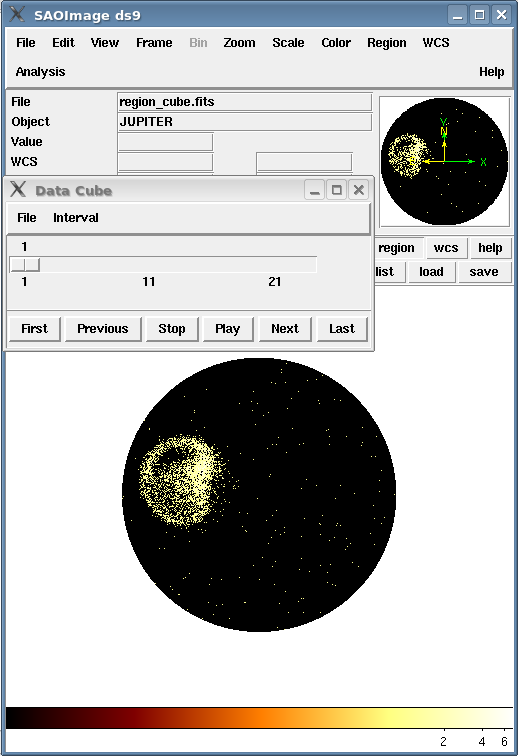### Figure 4: Region-filtered data cube

The spatial axes have been filtered by the region file, but the time axis is the same (23 intervals).

### Slicing a position-time data cube

It is also possible to slice time planes out of the file:

```unix% dmcopy "jupiter_cube.fits[#3=10][bin x,y]" plane10.fits
```

This filters the cube by selecting pixels where the logical axis 3 ("#3") coordinate is equal to 10.

The output file in an (x,y) image representing that slice of time:

```unix% dmlist plane10.fits cols

--------------------------------------------------------------------------------
Columns for Image Block EVENTS_IMAGE
--------------------------------------------------------------------------------

ColNo  Name                 Unit        Type             Range
1   EVENTS_IMAGE[500,500]              Int2(500x500)  -

--------------------------------------------------------------------------------
Physical Axis Transforms for Image Block EVENTS_IMAGE
--------------------------------------------------------------------------------

Group# Axis#
1   1,2    sky(x) = (+3900.0) +(+1.0)* ((#1)-(+0.50))
(y)   (+4100.0)  (+1.0)  ((#2) (+0.50))

--------------------------------------------------------------------------------
World Coordinate Axis Transforms for Image Block EVENTS_IMAGE
--------------------------------------------------------------------------------

Group# Axis#
1   1,2    EQPOS(RA ) = (+24.6832) +TAN[(-0.000136667)* (sky(x)-(+4096.50))]
(DEC)   (+8.6784 )      (+0.000136667)  (   (y) (+4096.50))
```

This is a two-dimensional image, shown in Figure 5.

### Figure 5: A time plane from the data cube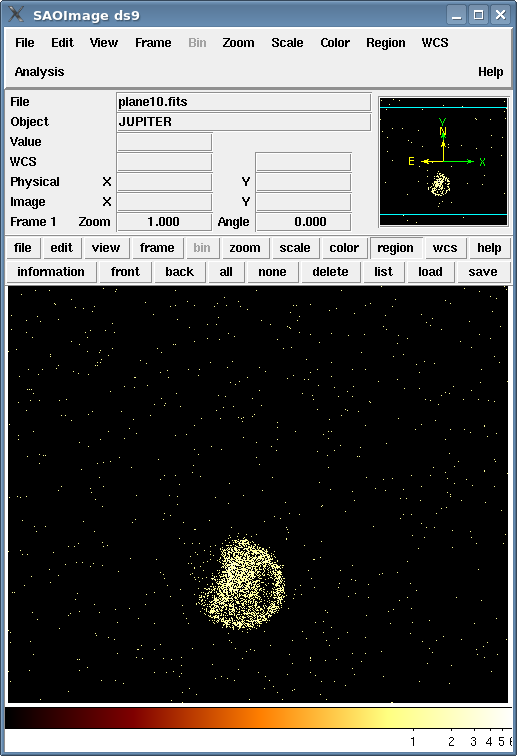### Figure 5: A time plane from the data cube

The time axis has been filtered to one interval and the spatial axes were binned by a factor of 1 into a 500x500 image.

## Removing Extra Axes: a 4D image that's really a 2D image

Some data, particularly radio images, have additional coordinate axes that are only one pixel wide, which are used to convey extra metadata. While useful, these extra axes may confuse applications that are designed for 2-dimensional data. dmcopy can be used to strip away the extra axes (at the cost of losing those metadata).

For instance, data obtained with the VLA looks like:

```unix% dmlist vla_radio.img cols

-----------------------------------------------------------------------
Columns for Image Block PRIMARY
-----------------------------------------------------------------------

ColNo  Name                 Unit        Type             Range
1   PRIMARY[256,256,1,1] JY/BEAM      Real4(256x256x1x1) -Inf:+Inf

-----------------------------------------------------------------------
Physical Axis Transforms for Image Block PRIMARY
-----------------------------------------------------------------------

Group# Axis#
1   1,2    POS(X) = (#1)
(Y)   (#2)
2   3      Z                    = #3
3   4      AXIS4                = #4

--------------------------------------------------------------------------------
World Coordinate Axis Transforms for Image Block PRIMARY
--------------------------------------------------------------------------------

Group# Axis#
1   1,2    EQPOS(RA ) = (+265.6222) +SIN[(-0.000277778)* (POS(X)-(+128.0))]
(DEC)   (-28.9884 )      (+0.000277778)  (   (Y) (+129.0))
2   3      FREQ                 = +4.33399E+10  +100000000.0 * (Z  -1.0)
3   4      STOKES               = AXIS4
```

This is a 256 x 256 x 1 x 1 image whose physical axes are known to CIAO as X, Y, Z, AXIS4 and whose world coordinate axes are called RA, DEC, FREQ and STOKES. If we want to make this into a simple RA, DEC image:

```unix% dmcopy "vla_radio.img[bin x,y]" vla_ra_dec.img
```

The file vla_ra_dec.img contains only the axes which were included in the binning specification; the additional information from the input file is discarded. Since we binned by a factor of one, the output image is also 256 x 256.

```unix% dmlist vla_ra_dec.img cols

--------------------------------------------------------------------------------
Columns for Image Block PRIMARY_IMAGE
--------------------------------------------------------------------------------

ColNo  Name                 Unit        Type             Range
1   PRIMARY_IMAGE[256,256]              Real4(256x256) -Inf:+Inf

--------------------------------------------------------------------------------
Physical Axis Transforms for Image Block PRIMARY_IMAGE
--------------------------------------------------------------------------------

Group# Axis#
1   1,2    POS(X) = (#1)
(Y)   (#2)

--------------------------------------------------------------------------------
World Coordinate Axis Transforms for Image Block PRIMARY_IMAGE
--------------------------------------------------------------------------------

Group# Axis#
1   1,2    EQPOS(RA ) = (+265.6222) +SIN[(-0.000277778)* (POS(X)-(+128.0))]
(DEC)   (-28.9884 )      (+0.000277778)  (   (Y) (+129.0))
```

Figure 6 shows the VLA image in ds9.

### Figure 6: VLA image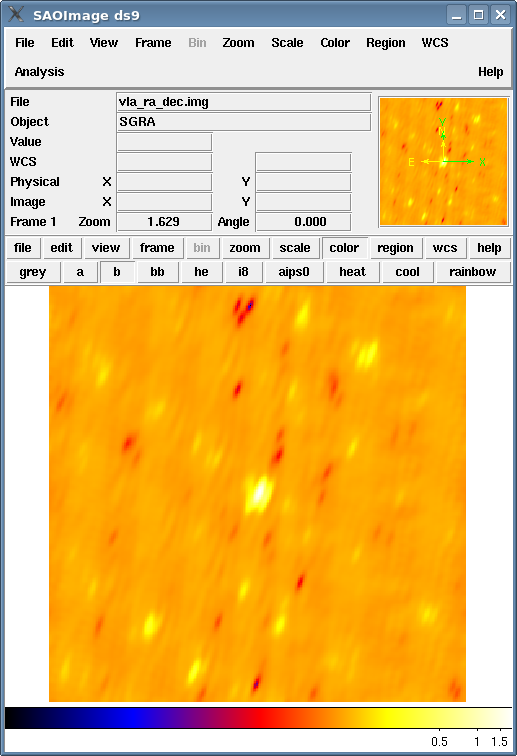### Figure 6: VLA image

The image was binned by a factor of one, so the output is still 256 x 256.

## History

 27 Jan 2006 new for CIAO 3.3: original version 01 Dec 2006 updated for CIAO 3.4: CIAO version 08 Jan 2008 updated for CIAO 4.0: changed filename in examples from jupiter.fits to acisf01463N002_evt2.fits 14 Jan 2009 updated for CIAO 4.1: images are inline 05 Feb 2010 reviewed for CIAO 4.2: no changes